# Metrics¶

All high-level functions have an optional metric argument that can be called to determine which metric is used in computing predictability.

Note

We use the phrase ‘observations’ o here to refer to the ‘truth’ data to which we compare the forecast f. These metrics can also be applied in reference to a control simulation, reconstruction, observations, etc. This would just change the resulting score from referencing skill to referencing potential predictability.

Internally, all metric functions require forecast and reference as inputs. The dimension dim is set internally by compute_hindcast() or compute_perfect_model() to specify over which dimensions the metric is applied. See Comparisons for more on the dim argument.

## Deterministic¶

Deterministic metrics assess the forecast as a definite prediction of the future, rather than in terms of probabilities. Another way to look at deterministic metrics is that they are a special case of probabilistic metrics where a value of one is assigned to one category and zero to all others [Jolliffe2011].

### Correlation Metrics¶

The below metrics rely fundamentally on correlations in their computation. In the literature, correlation metrics are typically referred to as the Anomaly Correlation Coefficient (ACC). This implies that anomalies in the forecast and observations are being correlated. Typically, this is computed using the linear Pearson Product-Moment Correlation. However, climpred also offers the Spearman’s Rank Correlation.

Note that the p value associated with these correlations is computed via a separate metric. Use pearson_r_p_value or spearman_r_p_value to compute p values assuming that all samples in the correlated time series are independent. Use pearson_r_eff_p_value or spearman_r_eff_p_value to account for autocorrelation in the time series by calculating the effective_sample_size.

#### Pearson Product-Moment Correlation Coefficient¶

# Enter any of the below keywords in metric=... for the compute functions.
In : print(f"\n\nKeywords: {metric_aliases['pearson_r']}")

Keywords: ['pearson_r', 'pr', 'acc', 'pacc']

climpred.metrics._pearson_r(forecast, reference, dim=None, **metric_kwargs)[source]

Pearson product-moment correlation coefficient.

A measure of the linear association between the forecast and observations that is independent of the mean and variance of the individual distributions. This is also known as the Anomaly Correlation Coefficient (ACC) when correlating anomalies.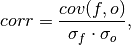where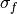and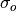represent the standard deviation of the forecast and observations over the experimental period, respectively.

Note

Use metric pearson_r_p_value or pearson_r_eff_p_value to get the corresponding p value.

Parameters: forecast (xarray object) – Forecast. reference (xarray object) – Reference (e.g. observations, control run). dim (str) – Dimension(s) to perform metric over. Automatically set by compute function. weights (xarray object, optional) – Weights to apply over dimension. Defaults to None. skipna (bool, optional) – If True, skip NaNs over dimension being applied to. Defaults to False.
Details:
 minimum -1.0 maximum 1.0 perfect 1.0 orientation positive

• xskillscore.pearson_r
• xskillscore.pearson_r_p_value
• climpred.pearson_r_p_value
• climpred.pearson_r_eff_p_value

#### Pearson Correlation p value¶

# Enter any of the below keywords in metric=... for the compute functions.
In : print(f"\n\nKeywords: {metric_aliases['pearson_r_p_value']}")

Keywords: ['pearson_r_p_value', 'p_pval', 'pvalue', 'pval']

climpred.metrics._pearson_r_p_value(forecast, reference, dim=None, **metric_kwargs)[source]

Probability that forecast and reference are linearly uncorrelated.

Two-tailed p value associated with the Pearson product-moment correlation coefficient (pearson_r), assuming that all samples are independent. Use pearson_r_eff_p_value to account for autocorrelation in the forecast and observations.

Parameters: forecast (xarray object) – Forecast. reference (xarray object) – Reference (e.g. observations, control run). dim (str) – Dimension(s) to perform metric over. Automatically set by compute function. weights (xarray object, optional) – Weights to apply over dimension. Defaults to None. skipna (bool, optional) – If True, skip NaNs over dimension being applied to. Defaults to False.
Details:
 minimum 0.0 maximum 1.0 perfect 1.0 orientation negative

• xskillscore.pearson_r
• xskillscore.pearson_r_p_value
• climpred.pearson_r
• climpred.pearson_r_eff_p_value

#### Effective Sample Size¶

# Enter any of the below keywords in metric=... for the compute functions.
In : print(f"\n\nKeywords: {metric_aliases['effective_sample_size']}")

Keywords: ['effective_sample_size', 'n_eff', 'eff_n']

climpred.metrics._effective_sample_size(forecast, reference, dim=None, **metric_kwargs)[source]

Effective sample size for temporally correlated data.

Note

Weights are not included here due to the dependence on temporal autocorrelation.

The effective sample size extracts the number of independent samples between two time series being correlated. This is derived by assessing the magnitude of the lag-1 autocorrelation coefficient in each of the time series being correlated. A higher autocorrelation induces a lower effective sample size which raises the correlation coefficient for a given p value.

The effective sample size is used in computing the effective p value. See pearson_r_eff_p_value and spearman_r_eff_p_value.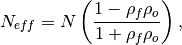where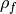and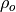are the lag-1 autocorrelation coefficients for the forecast and observations.

Parameters: forecast (xarray object) – Forecast. reference (xarray object) – Reference (e.g. observations, control run). dim (str) – Dimension(s) to perform metric over. Automatically set by compute function. skipna (bool, optional) – If True, skip NaNs over dimension being applied to. Defaults to False.
Details:
 minimum 0.0 maximum ∞ perfect N/A orientation positive

References

• Bretherton, Christopher S., et al. “The effective number of spatial degrees of freedom of a time-varying field.” Journal of climate 12.7 (1999): 1990-2009.

#### Pearson Correlation Effective p value¶

# Enter any of the below keywords in metric=... for the compute functions.
In : print(f"\n\nKeywords: {metric_aliases['pearson_r_eff_p_value']}")

Keywords: ['pearson_r_eff_p_value', 'p_pval_eff', 'pvalue_eff', 'pval_eff']

climpred.metrics._pearson_r_eff_p_value(forecast, reference, dim=None, **metric_kwargs)[source]

Probability that forecast and reference are linearly uncorrelated, accounting for autocorrelation.

Note

Weights are not included here due to the dependence on temporal autocorrelation.

The effective p value is computed by replacing the sample size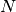in the t-statistic with the effective sample size,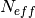. The same Pearson product-moment correlation coefficient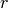is used as when computing the standard p value.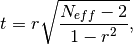whereis computed via the autocorrelation in the forecast and observations.whereandare the lag-1 autocorrelation coefficients for the forecast and observations.

Parameters: forecast (xarray object) – Forecast. reference (xarray object) – Reference (e.g. observations, control run). dim (str) – Dimension(s) to perform metric over. Automatically set by compute function. skipna (bool, optional) – If True, skip NaNs over dimension being applied to. Defaults to False.
Details:
 minimum 0.0 maximum 1.0 perfect 1.0 orientation negative

• climpred.effective_sample_size
• climpred.spearman_r_eff_p_value

References

• Bretherton, Christopher S., et al. “The effective number of spatial degrees of freedom of a time-varying field.” Journal of climate 12.7 (1999): 1990-2009.

#### Spearman’s Rank Correlation Coefficient¶

# Enter any of the below keywords in metric=... for the compute functions.
In : print(f"\n\nKeywords: {metric_aliases['spearman_r']}")

Keywords: ['spearman_r', 'sacc', 'sr']

climpred.metrics._spearman_r(forecast, reference, dim=None, **metric_kwargs)[source]

Spearman’s rank correlation coefficient.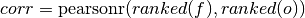This correlation coefficient is nonparametric and assesses how well the relationship between the forecast and observations can be described using a monotonic function. It is computed by first ranking the forecasts and observations, and then correlating those ranks using the pearson_r correlation.

This is also known as the anomaly correlation coefficient (ACC) when comparing anomalies, although the Pearson product-moment correlation coefficient (pearson_r) is typically used when computing the ACC.

Note

Use metric spearman_r_p_value or spearman_r_eff_p_value to get the corresponding p value.

Parameters: forecast (xarray object) – Forecast. reference (xarray object) – Reference (e.g. observations, control run). dim (str) – Dimension(s) to perform metric over. Automatically set by compute function. weights (xarray object, optional) – Weights to apply over dimension. Defaults to None. skipna (bool, optional) – If True, skip NaNs over dimension being applied to. Defaults to False.
Details:
 minimum -1.0 maximum 1.0 perfect 1.0 orientation positive

• xskillscore.spearman_r
• xskillscore.spearman_r_p_value
• climpred.spearman_r_p_value
• climpred.spearman_r_eff_p_value

#### Spearman’s Rank Correlation Coefficient p value¶

# Enter any of the below keywords in metric=... for the compute functions.
In : print(f"\n\nKeywords: {metric_aliases['spearman_r_p_value']}")

Keywords: ['spearman_r_p_value', 's_pval', 'spvalue', 'spval']

climpred.metrics._spearman_r_p_value(forecast, reference, dim=None, **metric_kwargs)[source]

Probability that forecast and reference are monotonically uncorrelated.

Two-tailed p value associated with the Spearman’s rank correlation coefficient (spearman_r), assuming that all samples are independent. Use spearman_r_eff_p_value to account for autocorrelation in the forecast and observations.

Parameters: forecast (xarray object) – Forecast. reference (xarray object) – Reference (e.g. observations, control run). dim (str) – Dimension(s) to perform metric over. Automatically set by compute function. weights (xarray object, optional) – Weights to apply over dimension. Defaults to None. skipna (bool, optional) – If True, skip NaNs over dimension being applied to. Defaults to False.
Details:
 minimum 0.0 maximum 1.0 perfect 1.0 orientation negative

• xskillscore.spearman_r
• xskillscore.spearman_r_p_value
• climpred.spearman_r
• climpred.spearman_r_eff_p_value

#### Spearman’s Rank Correlation Effective p value¶

# Enter any of the below keywords in metric=... for the compute functions.
In : print(f"\n\nKeywords: {metric_aliases['spearman_r_eff_p_value']}")

Keywords: ['spearman_r_eff_p_value', 's_pval_eff', 'spvalue_eff', 'spval_eff']

climpred.metrics._spearman_r_eff_p_value(forecast, reference, dim=None, **metric_kwargs)[source]

Probability that forecast and reference are monotonically uncorrelated, accounting for autocorrelation.

Note

Weights are not included here due to the dependence on temporal autocorrelation.

The effective p value is computed by replacing the sample sizein the t-statistic with the effective sample size,. The same Spearman’s rank correlation coefficientis used as when computing the standard p value.whereis computed via the autocorrelation in the forecast and observations.whereandare the lag-1 autocorrelation coefficients for the forecast and observations.

Parameters: forecast (xarray object) – Forecast. reference (xarray object) – Reference (e.g. observations, control run). dim (str) – Dimension(s) to perform metric over. Automatically set by compute function. skipna (bool, optional) – If True, skip NaNs over dimension being applied to. Defaults to False.
Details:
 minimum 0.0 maximum 1.0 perfect 1.0 orientation negative

• climpred.effective_sample_size
• climpred.pearson_r_eff_p_value

References

• Bretherton, Christopher S., et al. “The effective number of spatial degrees of freedom of a time-varying field.” Journal of climate 12.7 (1999): 1990-2009.

### Distance Metrics¶

This class of metrics simply measures the distance (or difference) between forecasted values and observed values.

#### Mean Squared Error (MSE)¶

# Enter any of the below keywords in metric=... for the compute functions.
In : print(f"\n\nKeywords: {metric_aliases['mse']}")

Keywords: ['mse']

climpred.metrics._mse(forecast, reference, dim=None, **metric_kwargs)[source]

Mean Sqaure Error (MSE).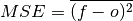The average of the squared difference between forecasts and observations. This incorporates both the variance and bias of the estimator. Because the error is squared, it is more sensitive to large forecast errors than mae, and thus a more conservative metric. For example, a single error of 2°C counts the same as two 1°C errors when using mae. On the other hand, the 2°C error counts double for mse. See Jolliffe and Stephenson, 2011.

Parameters: forecast (xarray object) – Forecast. reference (xarray object) – Reference (e.g. observations, control run). dim (str) – Dimension(s) to perform metric over. Automatically set by compute function. weights (xarray object, optional) – Weights to apply over dimension. Defaults to None. skipna (bool, optional) – If True, skip NaNs over dimension being applied to. Defaults to False.
Details:
 minimum 0.0 maximum ∞ perfect 0.0 orientation negative

• xskillscore.mse

References

• Ian T. Jolliffe and David B. Stephenson. Forecast Verification: A Practitioner’s Guide in Atmospheric Science. John Wiley & Sons, Ltd, Chichester, UK, December 2011. ISBN 978-1-119-96000-3 978-0-470-66071-3. URL: http://doi.wiley.com/10.1002/9781119960003.

#### Root Mean Square Error (RMSE)¶

# Enter any of the below keywords in metric=... for the compute functions.
In : print(f"\n\nKeywords: {metric_aliases['rmse']}")

Keywords: ['rmse']

climpred.metrics._rmse(forecast, reference, dim=None, **metric_kwargs)[source]

Root Mean Sqaure Error (RMSE).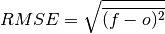The square root of the average of the squared differences between forecasts and observations.

Parameters: forecast (xarray object) – Forecast. reference (xarray object) – Reference (e.g. observations, control run). dim (str) – Dimension(s) to perform metric over. Automatically set by compute function. weights (xarray object, optional) – Weights to apply over dimension. Defaults to None. skipna (bool, optional) – If True, skip NaNs over dimension being applied to. Defaults to False.
Details:
 minimum 0.0 maximum ∞ perfect 0.0 orientation negative

• xskillscore.rmse

#### Mean Absolute Error (MAE)¶

# Enter any of the below keywords in metric=... for the compute functions.
In : print(f"\n\nKeywords: {metric_aliases['mae']}")

Keywords: ['mae']

climpred.metrics._mae(forecast, reference, dim=None, **metric_kwargs)[source]

Mean Absolute Error (MAE).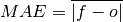The average of the absolute differences between forecasts and observations. A more robust measure of forecast accuracy than mse which is sensitive to large outlier forecast errors.

Parameters: forecast (xarray object) – Forecast. reference (xarray object) – Reference (e.g. observations, control run). dim (str) – Dimension(s) to perform metric over. Automatically set by compute function. weights (xarray object, optional) – Weights to apply over dimension. Defaults to None. skipna (bool, optional) – If True, skip NaNs over dimension being applied to. Defaults to False.
Details:
 minimum 0.0 maximum ∞ perfect 0.0 orientation negative

• xskillscore.mae

References

• Ian T. Jolliffe and David B. Stephenson. Forecast Verification: A Practitioner’s Guide in Atmospheric Science. John Wiley & Sons, Ltd, Chichester, UK, December 2011. ISBN 978-1-119-96000-3 978-0-470-66071-3. URL: http://doi.wiley.com/10.1002/9781119960003.

#### Median Absolute Error¶

# Enter any of the below keywords in metric=... for the compute functions.
In : print(f"\n\nKeywords: {metric_aliases['median_absolute_error']}")

Keywords: ['median_absolute_error']

climpred.metrics._median_absolute_error(forecast, reference, dim=None, **metric_kwargs)[source]

Median Absolute Error.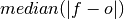The median of the absolute differences between forecasts and observations. Applying the median function to absolute error makes it more robust to outliers.

Parameters: forecast (xarray object) – Forecast. reference (xarray object) – Reference (e.g. observations, control run). dim (str) – Dimension(s) to perform metric over. Automatically set by compute function. skipna (bool, optional) – If True, skip NaNs over dimension being applied to. Defaults to False.
Details:
 minimum 0.0 maximum ∞ perfect 0.0 orientation negative

• xskillscore.median_absolute_error

### Normalized Distance Metrics¶

Distance metrics like mse can be normalized to 1. The normalization factor depends on the comparison type choosen. For example, the distance between an ensemble member and the ensemble mean is half the distance of an ensemble member with other ensemble members. See _get_norm_factor().

#### Normalized Mean Square Error (NMSE)¶

# Enter any of the below keywords in metric=... for the compute functions.
In : print(f"\n\nKeywords: {metric_aliases['nmse']}")

Keywords: ['nmse', 'nev']

climpred.metrics._nmse(forecast, reference, dim=None, **metric_kwargs)[source]

Normalized MSE (NMSE), also known as Normalized Ensemble Variance (NEV).

Mean Square Error (mse) normalized by the variance of the observations.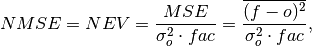whereis 1 when using comparisons involving the ensemble mean (m2e, e2c, e2r) and 2 when using comparisons involving individual ensemble members (m2c, m2m, m2r). See _get_norm_factor().

Note

climpred uses a single-valued internal reference forecast for the NMSE, in the terminology of Murphy 1988. I.e., we use a single climatological variance of the reference within the experimental window for normalizing MSE.

Parameters: forecast (xarray object) – Forecast. reference (xarray object) – Reference (e.g. observations, control run). dim (str) – Dimension(s) to perform metric over. Automatically set by compute function. weights (xarray object, optional) – Weights to apply over dimension. Defaults to None. skipna (bool, optional) – If True, skip NaNs over dimension being applied to. Defaults to False. comparison (str) – Name comparison needed for normalization factor fac, see _get_norm_factor() (Handled internally by the compute functions)
Details:
 minimum 0.0 maximum ∞ perfect 0.0 orientation negative better than climatology 0.0 - 1.0 worse than climatology > 1.0

References

• Griffies, S. M., and K. Bryan. “A Predictability Study of Simulated North Atlantic Multidecadal Variability.” Climate Dynamics 13, no. 7–8 (August 1, 1997): 459–87. https://doi.org/10/ch4kc4.
• Murphy, Allan H. “Skill Scores Based on the Mean Square Error and Their Relationships to the Correlation Coefficient.” Monthly Weather Review 116, no. 12 (December 1, 1988): 2417–24. https://doi.org/10/fc7mxd.

#### Normalized Mean Absolute Error (NMAE)¶

# Enter any of the below keywords in metric=... for the compute functions.
In : print(f"\n\nKeywords: {metric_aliases['nmae']}")

Keywords: ['nmae']

climpred.metrics._nmae(forecast, reference, dim=None, **metric_kwargs)[source]

Normalized Mean Absolute Error (NMAE).

Mean Absolute Error (mae) normalized by the standard deviation of the observations.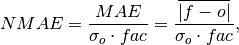whereis 1 when using comparisons involving the ensemble mean (m2e, e2c, e2r) and 2 when using comparisons involving individual ensemble members (m2c, m2m, m2r). See _get_norm_factor().

Note

climpred uses a single-valued internal reference forecast for the NMAE, in the terminology of Murphy 1988. I.e., we use a single climatological standard deviation of the reference within the experimental window for normalizing MAE.

Parameters: forecast (xarray object) – Forecast. reference (xarray object) – Reference (e.g. observations, control run). dim (str) – Dimension(s) to perform metric over. Automatically set by compute function. weights (xarray object, optional) – Weights to apply over dimension. Defaults to None. skipna (bool, optional) – If True, skip NaNs over dimension being applied to. Defaults to False. comparison (str) – Name comparison needed for normalization factor fac, see _get_norm_factor() (Handled internally by the compute functions)
Details:
 minimum 0.0 maximum ∞ perfect 0.0 orientation negative better than climatology 0.0 - 1.0 worse than climatology > 1.0

References

• Griffies, S. M., and K. Bryan. “A Predictability Study of Simulated North Atlantic Multidecadal Variability.” Climate Dynamics 13, no. 7–8 (August 1, 1997): 459–87. https://doi.org/10/ch4kc4.
• Murphy, Allan H. “Skill Scores Based on the Mean Square Error and Their Relationships to the Correlation Coefficient.” Monthly Weather Review 116, no. 12 (December 1, 1988): 2417–24. https://doi.org/10/fc7mxd.

#### Normalized Root Mean Square Error (NRMSE)¶

# Enter any of the below keywords in metric=... for the compute functions.
In : print(f"\n\nKeywords: {metric_aliases['nrmse']}")

Keywords: ['nrmse']

climpred.metrics._nrmse(forecast, reference, dim=None, **metric_kwargs)[source]

Normalized Root Mean Square Error (NRMSE).

Root Mean Square Error (rmse) normalized by the standard deviation of the observations.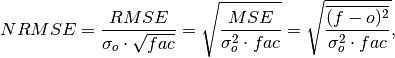whereis 1 when using comparisons involving the ensemble mean (m2e, e2c, e2r) and 2 when using comparisons involving individual ensemble members (m2c, m2m, m2r). See _get_norm_factor().

Note

climpred uses a single-valued internal reference forecast for the NRMSE, in the terminology of Murphy 1988. I.e., we use a single climatological variance of the reference within the experimental window for normalizing RMSE.

Parameters: forecast (xarray object) – Forecast. reference (xarray object) – Reference (e.g. observations, control run). dim (str) – Dimension(s) to perform metric over. Automatically set by compute function. weights (xarray object, optional) – Weights to apply over dimension. Defaults to None. skipna (bool, optional) – If True, skip NaNs over dimension being applied to. Defaults to False. comparison (str) – Name comparison needed for normalization factor fac, see _get_norm_factor() (Handled internally by the compute functions)
Details:
 minimum 0.0 maximum ∞ perfect 0.0 orientation negative better than climatology 0.0 - 1.0 worse than climatology > 1.0

References

• Bushuk, Mitchell, Rym Msadek, Michael Winton, Gabriel Vecchi, Xiaosong Yang, Anthony Rosati, and Rich Gudgel. “Regional Arctic Sea–Ice Prediction: Potential versus Operational Seasonal Forecast Skill.” Climate Dynamics, June 9, 2018. https://doi.org/10/gd7hfq.
• Hawkins, Ed, Steffen Tietsche, Jonathan J. Day, Nathanael Melia, Keith Haines, and Sarah Keeley. “Aspects of Designing and Evaluating Seasonal-to-Interannual Arctic Sea-Ice Prediction Systems.” Quarterly Journal of the Royal Meteorological Society 142, no. 695 (January 1, 2016): 672–83. https://doi.org/10/gfb3pn.
• Murphy, Allan H. “Skill Scores Based on the Mean Square Error and Their Relationships to the Correlation Coefficient.” Monthly Weather Review 116, no. 12 (December 1, 1988): 2417–24. https://doi.org/10/fc7mxd.

#### Mean Square Error Skill Score (MSESS)¶

# Enter any of the below keywords in metric=... for the compute functions.
In : print(f"\n\nKeywords: {metric_aliases['msess']}")

Keywords: ['msess', 'ppp', 'msss']

climpred.metrics._msess(forecast, reference, dim=None, **metric_kwargs)[source]

Mean Squared Error Skill Score (MSESS).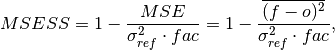whereis 1 when using comparisons involving the ensemble mean (m2e, e2c, e2r) and 2 when using comparisons involving individual ensemble members (m2c, m2m, m2r). See _get_norm_factor().

This skill score can be intepreted as a percentage improvement in accuracy. I.e., it can be multiplied by 100%.

Note

climpred uses a single-valued internal reference forecast for the MSSS, in the terminology of Murphy 1988. I.e., we use a single climatological variance of the reference within the experimental window for normalizing MSE.

Parameters: forecast (xarray object) – Forecast. reference (xarray object) – Reference (e.g. observations, control run). dim (str) – Dimension(s) to perform metric over. Automatically set by compute function. weights (xarray object, optional) – Weights to apply over dimension. Defaults to None. skipna (bool, optional) – If True, skip NaNs over dimension being applied to. Defaults to False. comparison (str) – Name comparison needed for normalization factor fac, see _get_norm_factor() (Handled internally by the compute functions)
Details:
 minimum -∞ maximum 1.0 perfect 1.0 orientation positive better than climatology > 0.0 equal to climatology 0.0 worse than climatology < 0.0

References

• Griffies, S. M., and K. Bryan. “A Predictability Study of Simulated North Atlantic Multidecadal Variability.” Climate Dynamics 13, no. 7–8 (August 1, 1997): 459–87. https://doi.org/10/ch4kc4.
• Murphy, Allan H. “Skill Scores Based on the Mean Square Error and Their Relationships to the Correlation Coefficient.” Monthly Weather Review 116, no. 12 (December 1, 1988): 2417–24. https://doi.org/10/fc7mxd.
• Pohlmann, Holger, Michael Botzet, Mojib Latif, Andreas Roesch, Martin Wild, and Peter Tschuck. “Estimating the Decadal Predictability of a Coupled AOGCM.” Journal of Climate 17, no. 22 (November 1, 2004): 4463–72. https://doi.org/10/d2qf62.
• Bushuk, Mitchell, Rym Msadek, Michael Winton, Gabriel Vecchi, Xiaosong Yang, Anthony Rosati, and Rich Gudgel. “Regional Arctic Sea–Ice Prediction: Potential versus Operational Seasonal Forecast Skill. Climate Dynamics, June 9, 2018. https://doi.org/10/gd7hfq.

#### Mean Absolute Percentage Error (MAPE)¶

# Enter any of the below keywords in metric=... for the compute functions.
In : print(f"\n\nKeywords: {metric_aliases['mape']}")

Keywords: ['mape']

climpred.metrics._mape(forecast, reference, dim=None, **metric_kwargs)[source]

Mean Absolute Percentage Error (MAPE).

Mean absolute error (mae) expressed as a percentage error relative to the observations.Parameters: forecast (xarray object) – Forecast. reference (xarray object) – Reference (e.g. observations, control run). dim (str) – Dimension(s) to perform metric over. Automatically set by compute function. weights (xarray object, optional) – Weights to apply over dimension. Defaults to None. skipna (bool, optional) – If True, skip NaNs over dimension being applied to. Defaults to False.
Details:
 minimum 0.0 maximum 1.0 perfect 0.0 orientation negative

• xskillscore.mape

#### Symmetric Mean Absolute Percentage Error (sMAPE)¶

# Enter any of the below keywords in metric=... for the compute functions.
In : print(f"\n\nKeywords: {metric_aliases['smape']}")

Keywords: ['smape']

climpred.metrics._smape(forecast, reference, dim=None, **metric_kwargs)[source]

Symmetric Mean Absolute Percentage Error (sMAPE).

Similar to the Mean Absolute Percentage Error (mape), but sums the forecast and observation mean in the denominator.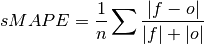Parameters: forecast (xarray object) – Forecast. reference (xarray object) – Reference (e.g. observations, control run). dim (str) – Dimension(s) to perform metric over. Automatically set by compute function. weights (xarray object, optional) – Weights to apply over dimension. Defaults to None. skipna (bool, optional) – If True, skip NaNs over dimension being applied to. Defaults to False.
Details:
 minimum 0.0 maximum 1.0 perfect 0.0 orientation negative

• xskillscore.smape

#### Unbiased Anomaly Correlation Coefficient (uACC)¶

# Enter any of the below keywords in metric=... for the compute functions.
In : print(f"\n\nKeywords: {metric_aliases['uacc']}")

Keywords: ['uacc']

climpred.metrics._uacc(forecast, reference, dim=None, **metric_kwargs)[source]

Bushuk’s unbiased Anomaly Correlation Coefficient (uACC).

This is typically used in perfect model studies. Because the perfect model Anomaly Correlation Coefficient (ACC) is strongly state dependent, a standard ACC (e.g. one computed using pearson_r) will be highly sensitive to the set of start dates chosen for the perfect model study. The Mean Square Skill Score (MSSS) can be related directly to the ACC as MSSS = ACC^(2) (see Murphy 1988 and Bushuk et al. 2019), so the unbiased ACC can be derived as uACC = sqrt(MSSS).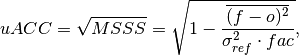whereis 1 when using comparisons involving the ensemble mean (m2e, e2c, e2r) and 2 when using comparisons involving individual ensemble members (m2c, m2m, m2r). See _get_norm_factor().

Note

Because of the square root involved, any negative MSSS values are automatically converted to NaNs.

Parameters: forecast (xarray object) – Forecast. reference (xarray object) – Reference (e.g. observations, control run). dim (str) – Dimension(s) to perform metric over. Automatically set by compute function. weights (xarray object, optional) – Weights to apply over dimension. Defaults to None. skipna (bool, optional) – If True, skip NaNs over dimension being applied to. Defaults to False. comparison (str) – Name comparison needed for normalization factor fac, see _get_norm_factor() (Handled internally by the compute functions)
Details:
 minimum 0.0 maximum 1.0 perfect 1.0 orientation positive better than climatology > 0.0 equal to climatology 0.0

References

• Bushuk, Mitchell, Rym Msadek, Michael Winton, Gabriel Vecchi, Xiaosong Yang, Anthony Rosati, and Rich Gudgel. “Regional Arctic Sea–Ice Prediction: Potential versus Operational Seasonal Forecast Skill.” Climate Dynamics, June 9, 2018. https://doi.org/10/gd7hfq.
• Allan H. Murphy. Skill Scores Based on the Mean Square Error and Their Relationships to the Correlation Coefficient. Monthly Weather Review, 116(12):2417–2424, December 1988. https://doi.org/10/fc7mxd.

### Murphy Decomposition Metrics¶

Metrics derived in [Murphy1988] which decompose the MSESS into a correlation term, a conditional bias term, and an unconditional bias term. See https://www-miklip.dkrz.de/about/murcss/ for a walk through of the decomposition.

#### Standard Ratio¶

# Enter any of the below keywords in metric=... for the compute functions.
In : print(f"\n\nKeywords: {metric_aliases['std_ratio']}")

Keywords: ['std_ratio']

climpred.metrics._std_ratio(forecast, reference, dim=None, **metric_kwargs)[source]

Ratio of standard deviations of the forecast over the reference.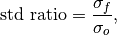whereandare the standard deviations of the forecast and the observations over the experimental period, respectively.

Parameters: forecast (xarray object) – Forecast. reference (xarray object) – Reference (e.g. observations, control run). dim (str) – Dimension(s) to perform metric over. Automatically set by compute functions.
Details:
 minimum 0.0 maximum ∞ perfect 1.0 orientation N/A

References

#### Conditional Bias¶

# Enter any of the below keywords in metric=... for the compute functions.
In : print(f"\n\nKeywords: {metric_aliases['conditional_bias']}")

Keywords: ['conditional_bias', 'c_b', 'cond_bias']

climpred.metrics._conditional_bias(forecast, reference, dim=None, **metric_kwargs)[source]

Conditional bias between forecast and reference.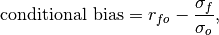whereandare the standard deviations of the forecast and observations over the experimental period, respectively.

Parameters: forecast (xarray object) – Forecast. reference (xarray object) – Reference (e.g. observations, control run). dim (str) – Dimension(s) to perform metric over. Automatically set by compute functions.
Details:
 minimum -∞ maximum 1.0 perfect 0.0 orientation negative

References

#### Unconditional Bias¶

# Enter any of the below keywords in metric=... for the compute functions.
In : print(f"\n\nKeywords: {metric_aliases['unconditional_bias']}")

Keywords: ['unconditional_bias', 'u_b', 'bias']


Simple bias of the forecast minus the observations.

climpred.metrics._unconditional_bias(forecast, reference, dim=None, **metric_kwargs)[source]

Unconditional bias.Parameters: forecast (xarray object) – Forecast. reference (xarray object) – Reference (e.g. observations, control run). dim (str) – Dimension(s) to perform metric over. Automatically set by compute functions.
Details:
 minimum -∞ maximum ∞ perfect 0.0 orientation negative

References

#### Bias Slope¶

# Enter any of the below keywords in metric=... for the compute functions.
In : print(f"\n\nKeywords: {metric_aliases['bias_slope']}")

Keywords: ['bias_slope']

climpred.metrics._bias_slope(forecast, reference, dim=None, **metric_kwargs)[source]

Bias slope between reference and forecast standard deviations.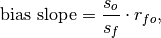where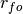is the Pearson product-moment correlation between the forecast and the observations and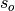and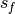are the standard deviations of the observations and forecast over the experimental period, respectively.

Parameters: forecast (xarray object) – Forecast. reference (xarray object) – Reference (e.g. observations, control run). dim (str) – Dimension(s) to perform metric over. Automatically set by compute functions.
Details:
 minimum 0.0 maximum ∞ perfect 1.0 orientation negative

References

#### Murphy’s Mean Square Error Skill Score¶

# Enter any of the below keywords in metric=... for the compute functions.
In : print(f"\n\nKeywords: {metric_aliases['msess_murphy']}")

Keywords: ['msess_murphy', 'msss_murphy']

climpred.metrics._msess_murphy(forecast, reference, dim=None, **metric_kwargs)[source]

Murphy’s Mean Square Error Skill Score (MSESS).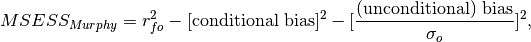where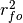represents the Pearson product-moment correlation coefficient between the forecast and observations andrepresents the standard deviation of the observations over the experimental period. See conditional_bias and unconditional_bias for their respective formulations.

Parameters: forecast (xarray object) – Forecast. reference (xarray object) – Reference (e.g. observations, control run). dim (str) – Dimension(s) to perform metric over. Automatically set by compute function. weights (xarray object, optional) – Weights to apply over dimension. Defaults to None. skipna (bool, optional) – If True, skip NaNs over dimension being applied to. Defaults to False.
Details:
 minimum -∞ maximum 1.0 perfect 1.0 orientation positive

• climpred.pearson_r
• climpred.conditional_bias
• climpred.unconditional_bias

References

## Probabilistic¶

Probabilistic metrics include the spread of the ensemble simulations in their calculations and assign a probability value between 0 and 1 to their forecasts [Jolliffe2011].

### Continuous Ranked Probability Score (CRPS)¶

# Enter any of the below keywords in metric=... for the compute functions.
In : print(f"\n\nKeywords: {metric_aliases['crps']}")

Keywords: ['crps']

climpred.metrics._crps(forecast, reference, **metric_kwargs)[source]

Continuous Ranked Probability Score (CRPS).

The CRPS can also be considered as the probabilistic Mean Absolute Error (mae). It compares the empirical distribution of an ensemble forecast to a scalar observation. Smaller scores indicate better skill.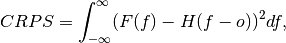where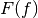is the cumulative distribution function (CDF) of the forecast (since the observations are not assigned a probability), and H() is the Heaviside step function where the value is 1 if the argument is positive (i.e., the forecast overestimates observations) or zero (i.e., the forecast equals observations) and is 0 otherwise (i.e., the forecast is less than observations).

Note

The CRPS is expressed in the same unit as the observed variable. It generalizes the Mean Absolute Error (MAE), and reduces to the MAE if the forecast is determinstic.

Parameters: forecast (xr.object) – Forecast with member dim. reference (xr.object) – References without member dim (e.g. observations, control run). metric_kwargs (xr.object) – If provided, the CRPS is calculated exactly with the assigned probability weights to each forecast. Weights should be positive, but do not need to be normalized. By default, each forecast is weighted equally.
Details:
 minimum 0.0 maximum ∞ perfect 0.0 orientation negative

References

• properscoring.crps_ensemble
• xskillscore.crps_ensemble

### Continuous Ranked Probability Skill Score (CRPSS)¶

# Enter any of the below keywords in metric=... for the compute functions.
In : print(f"\n\nKeywords: {metric_aliases['crpss']}")

Keywords: ['crpss']

climpred.metrics._crpss(forecast, reference, **metric_kwargs)[source]

Continuous Ranked Probability Skill Score.

This can be used to assess whether the ensemble spread is a useful measure for the forecast uncertainty by comparing the CRPS of the ensemble forecast to that of a reference forecast with the desired spread.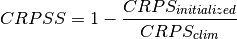Note

When assuming a Gaussian distribution of forecasts, use default gaussian=True. If not gaussian, you may specify the distribution type, xmin/xmax/tolerance for integration (see xskillscore.crps_quadrature).

Parameters: forecast (xr.object) – Forecast with member dim. reference (xr.object) – References without member dim. gaussian (bool, optional) – If True, assum Gaussian distribution for baseline skill. Defaults to True. cdf_or_dist (scipy.stats) – Function which returns the cumulative density of the forecast at value x. This can also be an object with a callable cdf() method such as a scipy.stats.distribution object. Defaults to scipy.stats.norm. xmin (float) – Lower bounds for integration. Only use if not assuming Gaussian. xmax (float) – tol (float, optional) – The desired accuracy of the CRPS. Larger values will speed up integration. If tol is set to None, bounds errors or integration tolerance errors will be ignored. Only use if not assuming Gaussian.
Details:
 minimum -∞ maximum 1.0 perfect 1.0 orientation positive better than climatology > 0.0 worse than climatology < 0.0

References

• Matheson, James E., and Robert L. Winkler. “Scoring Rules for Continuous Probability Distributions.” Management Science 22, no. 10 (June 1, 1976): 1087–96. https://doi.org/10/cwwt4g.
• Gneiting, Tilmann, and Adrian E Raftery. “Strictly Proper Scoring Rules, Prediction, and Estimation.” Journal of the American Statistical Association 102, no. 477 (March 1, 2007): 359–78. https://doi.org/10/c6758w.

Example

>>> compute_perfect_model(ds, control, metric='crpss')
>>> compute_perfect_model(ds, control, metric='crpss', gaussian=False,
cdf_or_dist=scipy.stats.norm, xminimum=-10,
xmaximum=10, tol=1e-6)


• properscoring.crps_ensemble
• xskillscore.crps_ensemble

### Continuous Ranked Probability Skill Score Ensemble Spread¶

# Enter any of the below keywords in metric=... for the compute functions.
In : print(f"\n\nKeywords: {metric_aliases['crpss_es']}")

Keywords: ['crpss_es']

climpred.metrics._crpss_es(forecast, reference, **metric_kwargs)[source]

Continuous Ranked Probability Skill Score Ensemble Spread.

If the ensemble variance is smaller than the observed mse, the ensemble is said to be under-dispersive (or overconfident). An ensemble with variance larger than the observations indicates one that is over-dispersive (underconfident).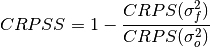Parameters: forecast (xr.object) – Forecast with member dim. reference (xr.object) – References without member dim. weights (xarray object, optional) – Weights to apply over dimension. Defaults to None. skipna (bool, optional) – If True, skip NaNs over dimension being applied to. Defaults to False.
Details:
 minimum -∞ maximum 0.0 perfect 0.0 orientation positive under-dispersive > 0.0 over-dispersive < 0.0

References

• Kadow, Christopher, Sebastian Illing, Oliver Kunst, Henning W. Rust, Holger Pohlmann, Wolfgang A. Müller, and Ulrich Cubasch. “Evaluation of Forecasts by Accuracy and Spread in the MiKlip Decadal Climate Prediction System.” Meteorologische Zeitschrift, December 21, 2016, 631–43. https://doi.org/10/f9jrhw.
Range:
• perfect: 0
• else: negative

### Brier Score¶

# Enter any of the below keywords in metric=... for the compute functions.
In : print(f"\n\nKeywords: {metric_aliases['brier_score']}")

Keywords: ['brier_score', 'brier', 'bs']

climpred.metrics._brier_score(forecast, reference, **metric_kwargs)[source]

Brier Score.

The Mean Square Error (mse) of probabilistic two-category forecasts where the observations are either 0 (no occurrence) or 1 (occurrence) and forecast probability may be arbitrarily distributed between occurrence and non-occurrence. The Brier Score equals zero for perfect (single-valued) forecasts and one for forecasts that are always incorrect.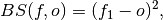where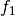is the forecast probability of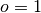.

Note

The Brier Score requires that the reference is binary, i.e., can be described as one (a “hit”) or zero (a “miss”).

Parameters: forecast (xr.object) – Forecast with member dim. reference (xr.object) – References without member dim. func (function) – Function to be applied to reference and forecasts and then mean('member') to get forecasts and reference in interval [0,1].
Details:
 minimum 0.0 maximum 1.0 perfect 0.0 orientation negative
Reference:

• properscoring.brier_score
• xskillscore.brier_score

Example

>>> def pos(x): return x > 0
>>> compute_perfect_model(ds, control, metric='brier_score', func=pos)


### Threshold Brier Score¶

# Enter any of the below keywords in metric=... for the compute functions.
In : print(f"\n\nKeywords: {metric_aliases['threshold_brier_score']}")

Keywords: ['threshold_brier_score', 'tbs']

climpred.metrics._threshold_brier_score(forecast, reference, **metric_kwargs)[source]

Brier score of an ensemble for exceeding given thresholds.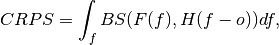where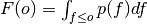is the cumulative distribution function (CDF) of the forecast distribution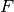,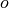is a point estimate of the true observation (observational error is neglected),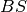denotes the Brier score and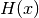denotes the Heaviside step function, which we define here as equal to 1 for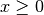and 0 otherwise.

Parameters: forecast (xr.object) – Forecast with member dim. reference (xr.object) – References without member dim. threshold (int, float, xr.object) – Threshold to check exceedance, see properscoring.threshold_brier_score.
Details:
 minimum 0.0 maximum 1.0 perfect 0.0 orientation negative

References

• properscoring.threshold_brier_score
• xskillscore.threshold_brier_score

Example

>>> compute_perfect_model(ds, control,
metric='threshold_brier_score', threshold=.5)


## User-defined metrics¶

You can also construct your own metrics via the climpred.metrics.Metric class.

 Metric(name, function, positive, …[, …]) Master class for all metrics.

First, write your own metric function, similar to the existing ones with required arguments forecast, reference, dim=None, and **metric_kwargs:

from climpred.metrics import Metric

def _my_msle(forecast, reference, dim=None, **metric_kwargs):
"""Mean squared logarithmic error (MSLE).
https://peltarion.com/knowledge-center/documentation/modeling-view/build-an-ai-model/loss-functions/mean-squared-logarithmic-error."""
return ( (np.log(forecast + 1) + np.log(reference + 1) ) ** 2).mean(dim)


Then initialize this metric function with climpred.metrics.Metric:

_my_msle = Metric(
name='my_msle',
function=_my_msle,
probabilistic=False,
positive=False,
unit_power=0,
)


Finally, compute skill based on your own metric:

skill = compute_perfect_model(ds, control, metric=_my_msle)


Once you come up with an useful metric for your problem, consider contributing this metric to climpred, so all users can benefit from your metric, see contributing.